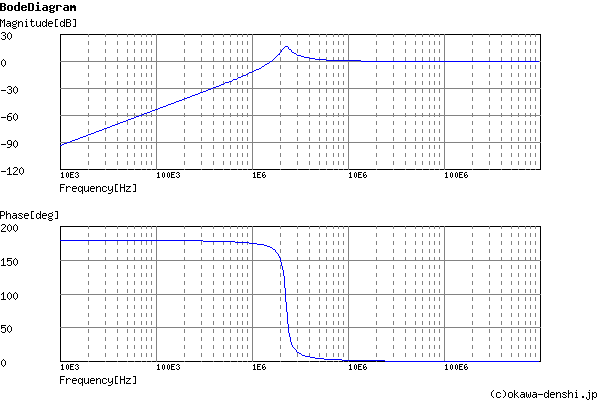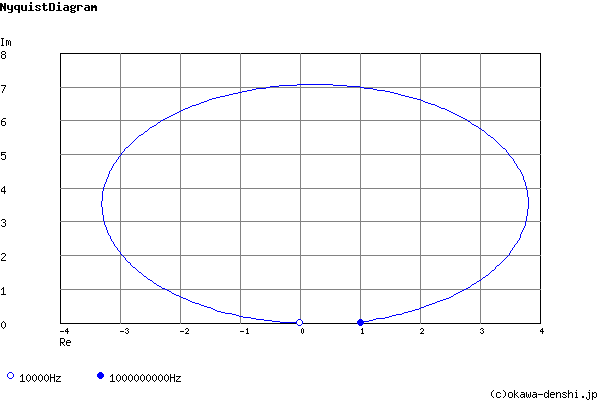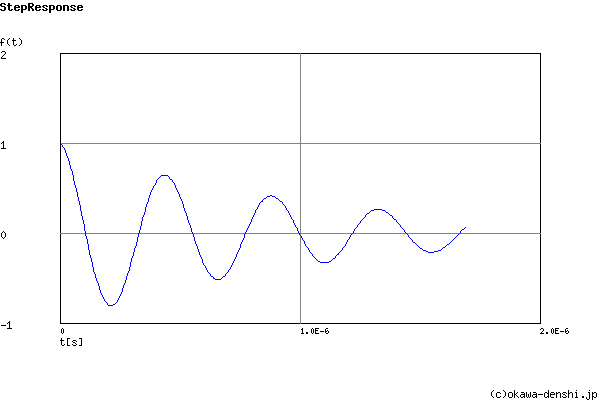# (Sample)RLC High-pass Filter Design Tool - Result -

Calculated the transfer function for the RLC High-pass filter, displayed on graphs, showing Bode diagram, Nyquist diagram, Impulse response and Step response.

## RLC Filter

 Vin(s)→→Vout(s)
(Sample)Transfer Function:
 G(s)= s2 s2+2000000s+2E+14

#### Cut-off frequency

fc = 2250790.79039[Hz]

#### Quality factor

Q = 7.07106781187

#### Damping ratio

ζ = 0.0707106781187

#### Pole(s)

p = -159154.943092 +2245156.76206i[Hz]
|p|= 2250790.79039[Hz]
p = -159154.943092-2245156.76206i[Hz]
|p|= 2250790.79039[Hz]

z = 0[Hz]
|z|= 0[Hz]
z = -0[Hz]
|z|= 0[Hz]

#### Phase margin

pm= NAN[deg] (f =0[Hz])

#### Oscillation frequency

f = 2245156.76206[Hz]

#### Overshoot (in absolute value)

The 1st peak  gpk = -0.81 (t =2.1E-07[sec])
The 2nd peak  gpk = 0.65 (t =4.3E-07[sec])
The 3rd peak  gpk = -0.52 (t =6.6E-07[sec])

#### Final value of the step response (on the condition that the system converged when t goes to infinity)

g(∞) = 0

 R=Ω L=H C=F
p:pico, n:nano, u:micro, k:kilo, M:mega

### Frequency analysis

Bode diagram
Phase  Group delay
Nyquist diagram
Pole, zero
Phase margin
Oscillation analysis
Analysis on frequency range:
f1=∼f2=[Hz] (optional)

### Transient analysis

Step response
Impulse response
Overshoot
Final value of the step response
Analysis on time range:
0∼[sec] (optional)

# Frequency analysis# Transient analysis